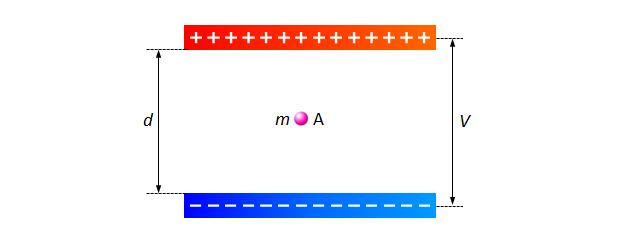# Charged particle between 2 charged platesAs shown above, a charged particle $A$ with mass $m$ is placed between two parallel charged plates parallel to the ground with the negatively charged plate closer to the ground. The distance between the two plates is $d$ and the electric potential difference between the two plates is $V$. If the particle is stationary, then what is the magnitude of the quantity of the electric charge of $A?$

Note: $g$ denotes the gravitational acceleration.

×